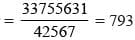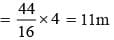# Olympiad Test : Number System - 1

## 10 Questions MCQ Test Maths Olympiad Class 6 | Olympiad Test : Number System - 1

Description
Attempt Olympiad Test : Number System - 1 | 10 questions in 20 minutes | Mock test for Class 6 preparation | Free important questions MCQ to study Maths Olympiad Class 6 for Class 6 Exam | Download free PDF with solutions
QUESTION: 1

Solution:
QUESTION: 2

Solution:
QUESTION: 3

### What is the value of CLXVI?

Solution:

CLXVI = 100 + 50 + 10 + 5 + 1 = 166

QUESTION: 4

The product of two numbers is 33755631. If one number is 42567, then what is the other number?

Solution:

Other number =QUESTION: 5

What is the nearest thousand of the sum 21297 + 27867 + 42679 ?

Solution:

21297 + 27867 + 42679
= 21000 + 28000 + 43000
= 92000

QUESTION: 6

What is the round off value of the product 43 and 78 ?

Solution:

43 × 78
= 40 × 80  = 3200

QUESTION: 7

What number must be subtracted from 1101010 to get 336414 ?

Solution:

1101010 – 336414
= 764596

QUESTION: 8

The population of a town in the year 2010 was 14693675. In the following year, the population became 18002403. What is the increase in the population?

Solution:

Increase in population = 18002403 – 14693675
= 3308728

QUESTION: 9

In the given numbers which will exactly come in the middle?

3307, 3279, 3467, 3502, 3379, 3667, 3287

Solution:

Numbers in ascending order are 3279, 3287, 3307, (3379), 3467, 3502, 3667.

QUESTION: 10

For making 16 shirts 44 metres of cloth is needed. How much cloth is required for 4 shirts?

Solution:

Cloth required for 4 shirtsUse Code STAYHOME200 and get INR 200 additional OFF Use Coupon Code